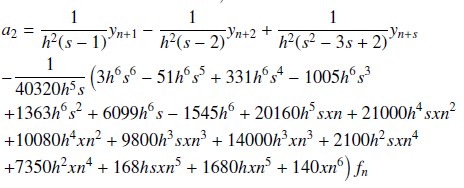# Solving third order ordinary differential equations directly using hybrid numerical models

• J. O. Kuboye Department of Mathematics, Federal University Oye-Ekiti, Nigeria
• O. F. Quadri Department of Mathematics, Federal University Oye-Ekiti, Nigeria
• O. R. Elusakin Department of Mathematics, Federal University Oye-Ekiti, Nigeria
Keywords: Interpolation, collocation, block method, multistep method and third order initial value problem

### Abstract

In this work, numerical methods for solving third order initial value problems of ordinary differential equations are developed. Multi-step collocation is used in deriving the methods, where power series approximate solution is employed as a basis function. Gaussian elimination approach is considered in finding the unknown variables \$a_j, j=0(1)8\$ in interpolation and collocation equations, which are substituted into the power series to give the continuous implicit schemes. The discrete schemes and its derivatives are derived by evaluating the grid and non-grid points. These schemes are arranged in a matrix form to produce block methods. The order of the developed methods are found to be six. The numerical results proved the efficiency of the methods over the existing methods.

### References

D. A. Awoyemi, ``Class of continuous methods for general second order initial value problems in ordinary differential equations", International Journal of Computer Mathematics 72 (2009) 29.

S. O. Fatunla, Numerical methods for initial value problems in ordinary differential equations, Academic press Inc. Harcourt Brace Jovanovich Publishers, New York (1988) DOI: https://doi.org/10.1016/B978-0-12-249930-2.50012-6

bibitem{3} P. Henrici, ``Discrete variable methods in Ordinary Differential Equations", New York, NY; Wiley 571 (1962) 419.

J. O. Kuboye & Z. Omar, ``Numerical solution of third order ordinary differential equations using a seven-step block method", International Journal of Mathematical Analysis 9 (2015) 743.

J. D. Lambert, ``Computational Method in Ordinary Differential Equation", John Wiley and Sons, Inc 91 (1973) 288.

B. T. Olabode, ``A six-step scheme for the solution of fourth order ordinary differential equation", The Pacific Journal of Science and Technology 10 (2009) 143.

B. T. Olabode, ``Block multistep method for third order ordinary differential equations", FUTA Journal of Research in Sciences 2 (2013) 194.

Z. Omar & M. Suleiman, ``Solving higher order odes directly using parallel 2-point explicit block method", Matematika. Pengintegrasian Teknologi Dalam Sains Matematik. Universiti Sains Malaysia 21 (2005) 15.

A. M. Sagir, ``On the Approximate Solution of Continuous Coefficients for Solving Third Order Ordinary Differential Equations", International Journal of Mathematical, Computational, Physical, Electrical and Computer Engineering 8 (2018) 67.

J. Sunday, ``On the Oscillation Criteria and Computation of Third Order Oscillatory Differential Equations", Communications in Mathematics and Applications 9 (2018) 615.Published
2020-05-13
How to Cite
J. O. Kuboye, O. F. Quadri, & O. R. Elusakin. (2020). Solving third order ordinary differential equations directly using hybrid numerical models. Journal of the Nigerian Society of Physical Sciences, 2(2), 69-76. https://doi.org/10.46481/jnsps.2020.43
Section
Original Research